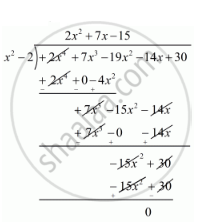# Find All Zeros of the Polynomial 2x4 + 7x3 − 19x2 − 14x + 30, If Two of Its Zeros Are Sqrt2 and -sqrt2. - Mathematics

#### Question

Find all zeros of the polynomial 2x4 + 7x3 − 19x2 − 14x + 30, if two of its zeros are sqrt2 and -sqrt2.

#### Solution

We know that if x = a is a zero of a polynomial, then x - a is a factor of f(x).

Since, sqrt2 and -sqrt2 are zeros of f(x).

Therefore

(x+sqrt2)(x-sqrt2)=x^2-(sqrt2)^2

= x2 - 2

x2 - 2 is a factor of f(x). Now, we divide 2x4 + 7x3 − 19x2 − 14x + 30 by g(x) = x2 - 2 to find the zero of f(x).By using division algorithm we have

f(x) = g(x) x q(x) - r(x)

2x4 + 7x3 − 19x2 − 14x + 30 = (x2 - 2)(2x2 + 7x - 15) + 0

2x4 + 7x3 − 19x2 − 14x + 30 =(x+sqrt2)(x-sqrt2)(2x^2+10x-3x-15)

2x4 + 7x3 − 19x2 − 14x + 30 =(x+sqrt2)(x-sqrt2)[2x(x+5)-3(x+5)]

2x4 + 7x3 − 19x2 − 14x + 30 =(x+sqrt2)(x-sqrt2)(2x-3)(x+5)

Hence, the zeros of the given polynomial are -sqrt2, +sqrt2,  (+3)/2, -5.

Is there an error in this question or solution?

#### APPEARS IN

RD Sharma Solution for Class 10 Maths (2018 (Latest))
Chapter 2: Polynomials
2.3 | Q: 10 | Page no. 57

#### Video TutorialsVIEW ALL 

Find All Zeros of the Polynomial 2x4 + 7x3 − 19x2 − 14x + 30, If Two of Its Zeros Are Sqrt2 and -sqrt2. Concept: Division Algorithm for Polynomials.